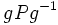# Sylow implies order-conjugate

This article gives the statement and possibly, proof, of an implication relation between two subgroup properties. That is, it states that every subgroup satisfying the first subgroup property (i.e., Sylow subgroup) must also satisfy the second subgroup property (i.e., order-conjugate subgroup)
View all subgroup property implications | View all subgroup property non-implications

## Statement

### Statement with symbols

There are two formulations:

• Any two$p$-Sylow subgroups of a finite group are conjugate subgroups.
• Suppose$P$ is a$p$-Sylow subgroup of a finite group$G$, and$Q$ is a subgroup of$G$ of the same order as$P$. Then$P$ and$Q$ are conjugate in$G$.

The equivalence of the formulations follows from the fact that the condition of being a$p$-Sylow subgroup is completely determined by the order.

## Related facts

### Other parts of Sylow's theorem

All these facts together are known as Sylow's theorem.

## Facts used

1. Sylow implies order-dominating: This states that if$P$ is$p$-Sylow and$Q$ is a$p$-subgroup, there exists a conjugate$gPg^{-1}$ of$P$ such that$Q \le gPg^{-1}$.
2. Order-dominating implies order-conjugate in finite

## Proof

### Proof using order-domination

This proof follows directly from facts (1) and (2).